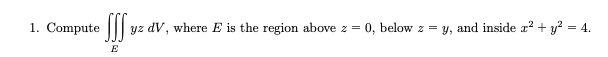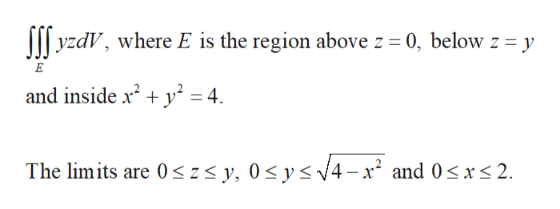# 1. Computeyyz dV, where E is the region above z = 0, below z = y, and inside r2 + y2 = 4E

Question
27 views

1. Compute  E yz dV , where E is the region above z  0, below z  y, and inside x 2 y 2  4help_outlineImage Transcriptionclose1. Computey yz dV, where E is the region above z = 0, below z = y, and inside r2 + y2 = 4 E fullscreen
check_circle

Step 1

Obtain the limit of integration as follows.help_outlineImage TranscriptioncloseyzdV, where E is the region above z= 0, below z = y E and inside x2 y2= 4 The limits are 0szs y, 0 sys4-x2 and 0s xs 2 fullscreen
Step 2

Evaluate the integrati...

### Want to see the full answer?

See Solution

#### Want to see this answer and more?

Solutions are written by subject experts who are available 24/7. Questions are typically answered within 1 hour.*

See Solution
*Response times may vary by subject and question.
Tagged in

### Math